Question

# Term Description Risk A. The term applied to the risk of an asset that is measured...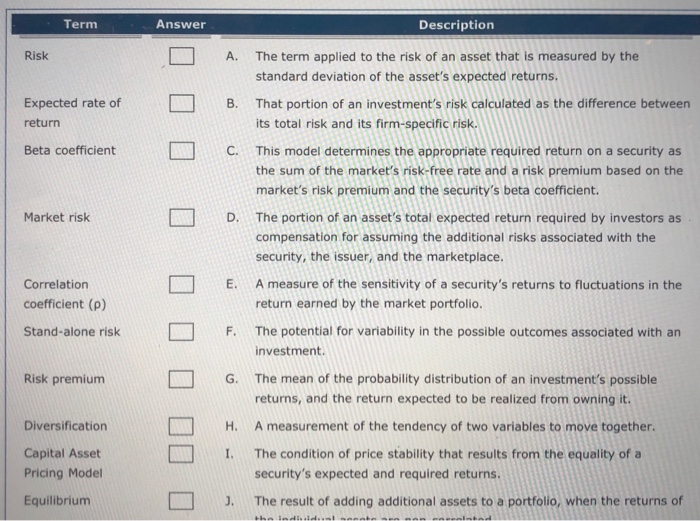Term Description Risk A. The term applied to the risk of an asset that is measured by the standard deviation of the asset's expected returns. That portion of an investment's risk calculated as the difference between its total risk and its firm-specific risk. Expected rate of return B. Beta coefficient L C . This model determines the appropriate required return on a security as the sum of the market's risk-free rate and a risk premium based on the market's risk premium and the security's beta coefficient. Market risk D. 1000 0 00000 0 The portion of an asset's total expected return required by investors as compensation for assuming the additional risks associated with the security, the issuer, and the marketplace. Correlation coefficient (P) A measure of the sensitivity of a security's returns to fluctuations in the return earned by the market portfolio. Stand-alone risk F. The potential for variability in the possible outcomes associated with an investment. Risk premium Diversification G. The mean of the probability distribution of an investment's possible returns, and the return expected to be realized from owning it. H. A measurement of the tendency of two variables to move together. I. The condition of price stability that results from the equality of a security's expected and required returns. J. The result of adding additional assets to a portfolio, when the returns of Capital Asset Pricing Model Equilibrium the ladiel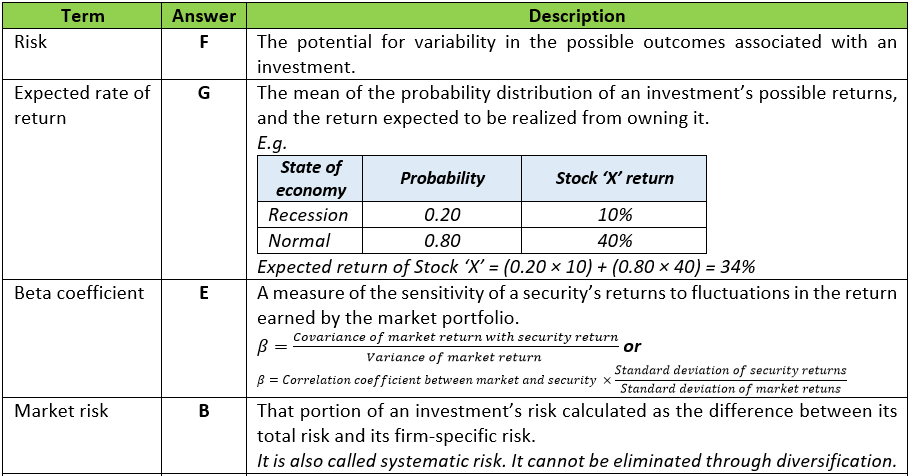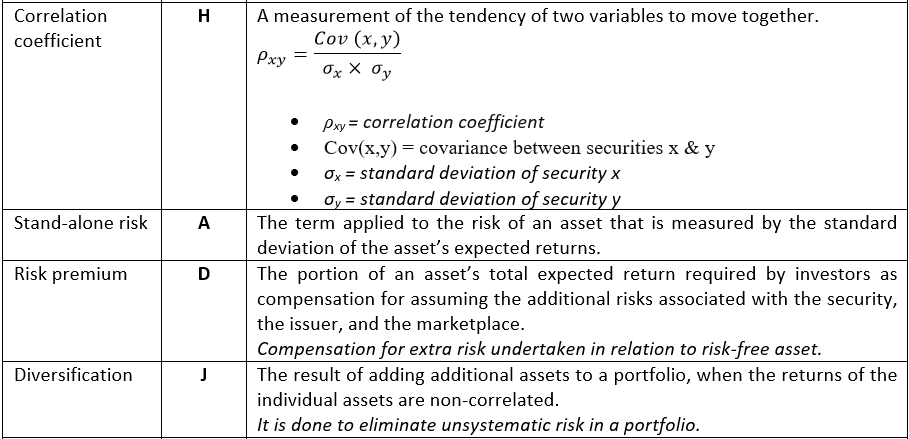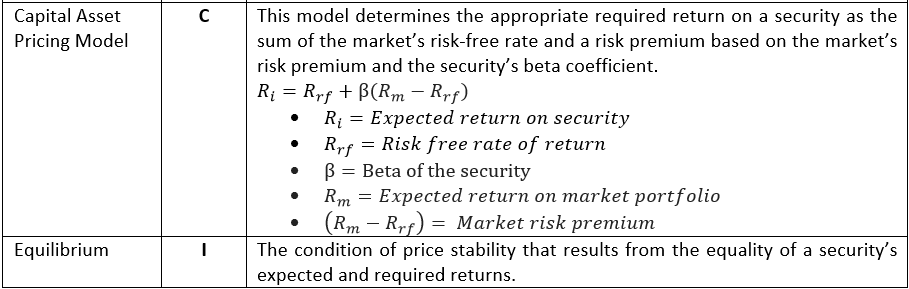#### Earn Coins

Coins can be redeemed for fabulous gifts.

Similar Homework Help Questions
• ### Term Answer Description Risk A. The potential for variability in the possible outcomes associated with an...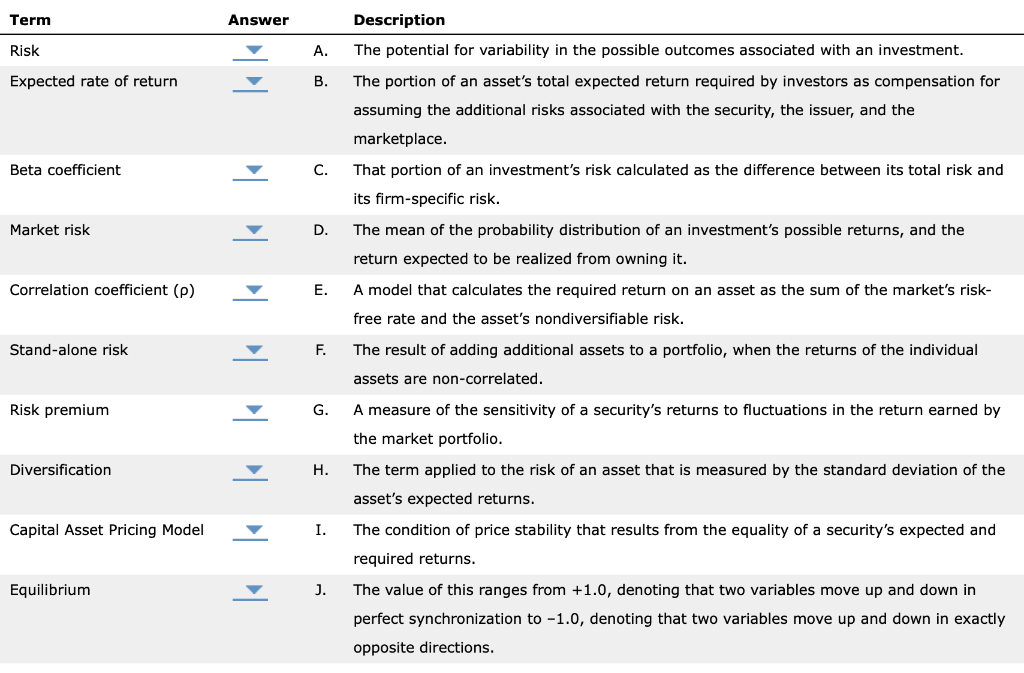Term Answer Description Risk A. The potential for variability in the possible outcomes associated with an investment. Expected rate of return The portion of an asset's total expected return required by investors as compensation for assuming the additional risks associated with the security, the issuer, and the marketplace. Beta coefficient That portion of an investment's risk calculated as the difference between its total risk and its firm-specific risk. Market risk The mean of the probability distribution of an investment's possible...

• ### Term Answer Description Risk A. The risk of an asset when it is the only asset...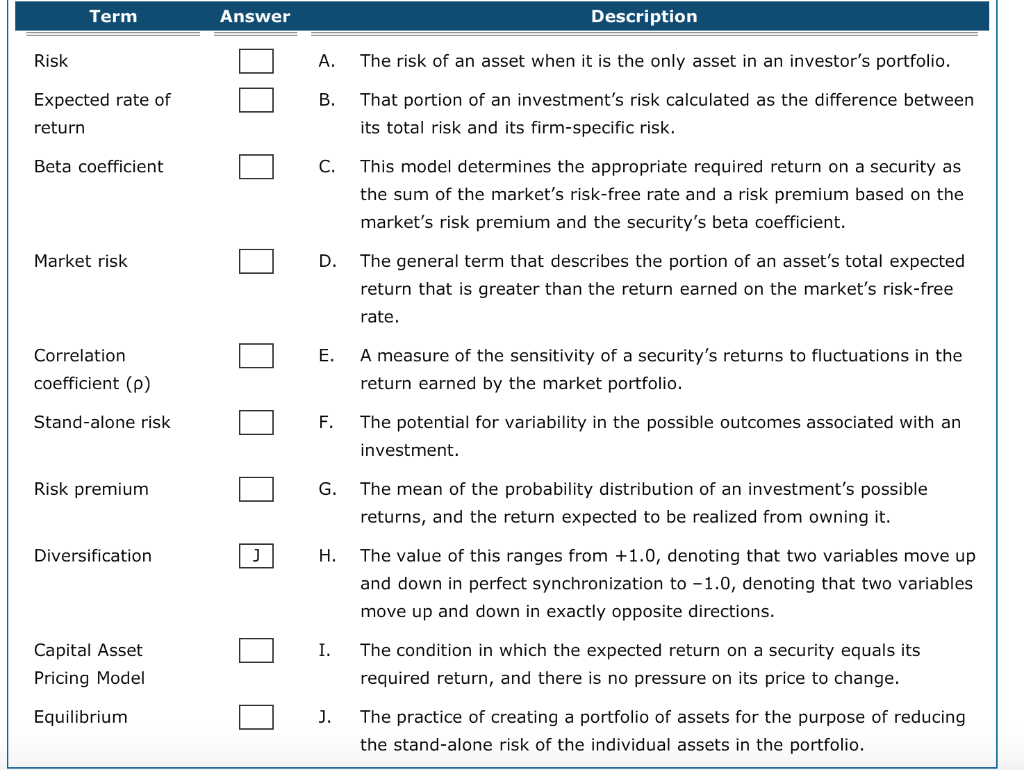Term Answer Description Risk A. The risk of an asset when it is the only asset in an investor's portfolio. Expected rate of return That portion of an investment's risk calculated as the difference between its total risk and its firm-specific risk. Beta coefficient This model determines the appropriate required return on a security as the sum of the market's risk-free rate and a risk premium based on the market's risk premium and the security's beta coefficient. Market risk The...

• ### Ch 02: Assignment - Risk and Return: Part 1 Term Answer Risk A Expected rate of...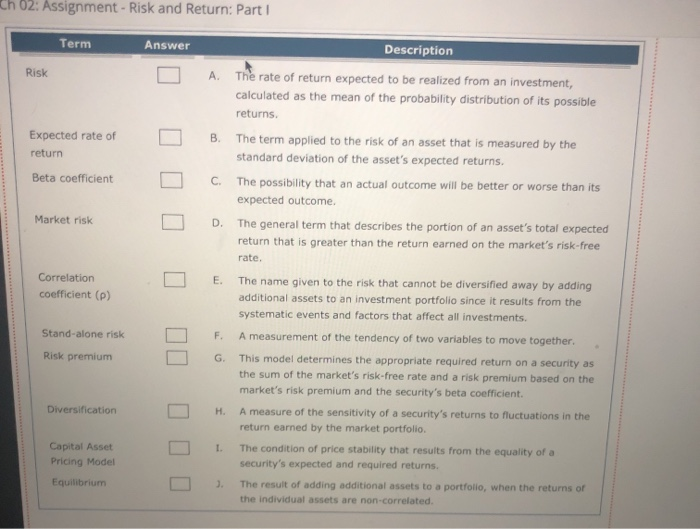Ch 02: Assignment - Risk and Return: Part 1 Term Answer Risk A Expected rate of return B Description The rate of return expected to be realized from an investment, calculated as the mean of the probability distribution of its possible returns. The term applied to the risk of an asset that is measured by the standard deviation of the asset's expected returns. The possibility that an actual outcome will be better or worse than its expected outcome The general...

• ### Term Answer Description A. The general term that describes the portion of an asset's total expected...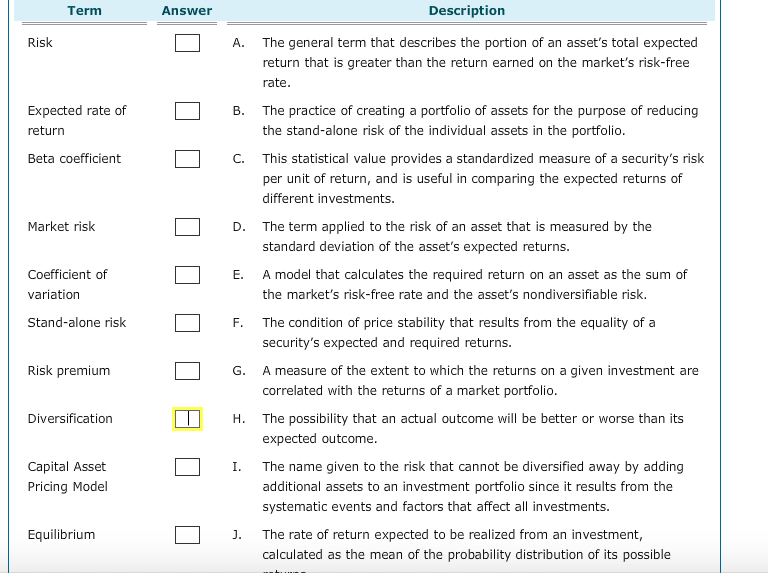Term Answer Description A. The general term that describes the portion of an asset's total expected return that is greater than the return earned on the market's risk-free rate Expected rate of return B. The practice of creating a portfolio of assets for the purpose of reducing the stand-alone risk of the individual assets in the portfolio Beta coefficient C. This statistical value provides a standardized measure of a security's risk per unit of return, and is useful in comparing...

• ### 2. 3: Risk and Rates of Return: Risk in Portfolio Context Risk and Rates of Return:...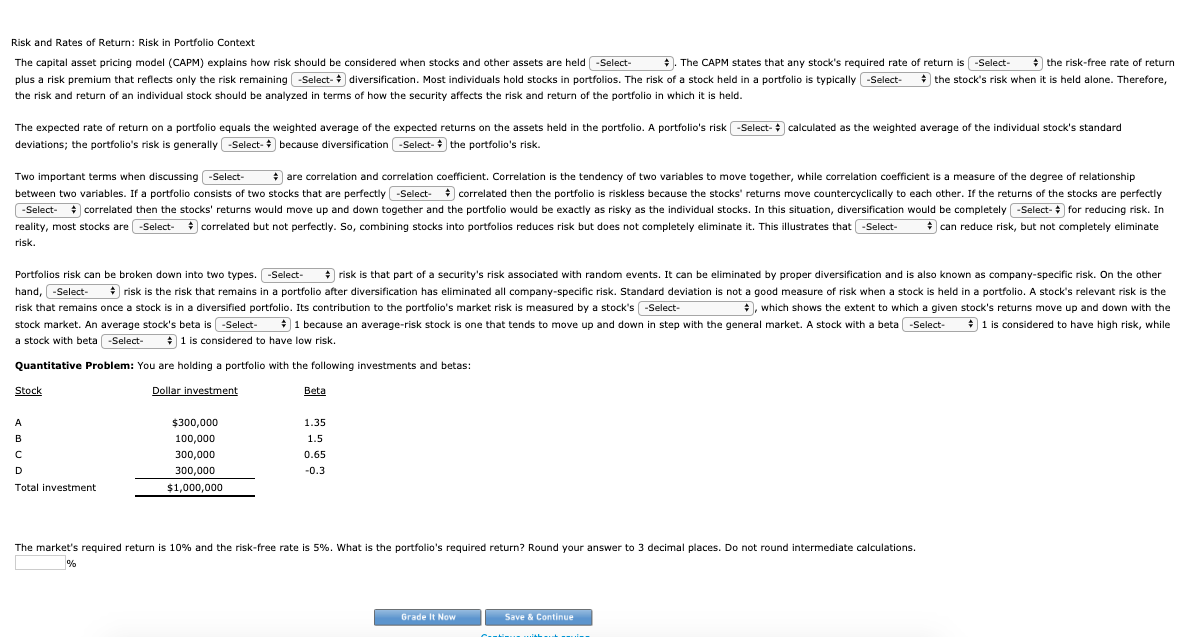2. 3: Risk and Rates of Return: Risk in Portfolio Context Risk and Rates of Return: Risk in Portfolio Context The capital asset pricing model (CAPM) explains how risk should be considered when stocks and other assets are held . The CAPM states that any stock's required rate of return is the risk-free rate of return plus a risk premium that reflects only the risk remaining diversification. Most individuals hold stocks in portfolios. The risk of a stock held in...

• ### Asset Y has a beta of 1.2. The risk-free rate of return is 6 percent, while...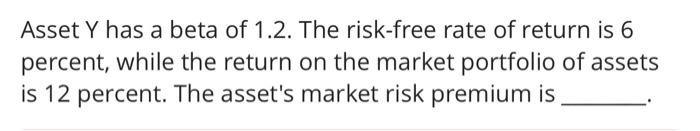Asset Y has a beta of 1.2. The risk-free rate of return is 6 percent, while the return on the market portfolio of assets is 12 percent. The asset's market risk premium is Asset Y has a beta of 1.2. The risk-free rate of return is 6 percent, while the return on the market portfolio of assets is 12 percent. The asset's market risk premium is

• ### od The capital asset pricing model (CAPM) explains how risk should be considered when stocks and...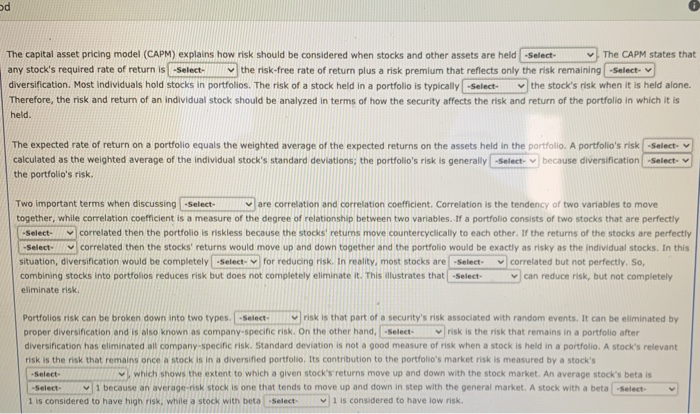od The capital asset pricing model (CAPM) explains how risk should be considered when stocks and other assets are held -Select- The CAPM states that any stock's required rate of return is -Select the risk-free rate of return plus a risk premium that reflects only the risk remaining -Select- diversification. Most individuals hold stocks in portfolios. The risk of a stock held in a portfolio is typically -Select the stock's risk when it is held alone. Therefore, the risk and...

• ### The capital asset pricing model: applies to portfolios but not too individual securities. rewards investors based...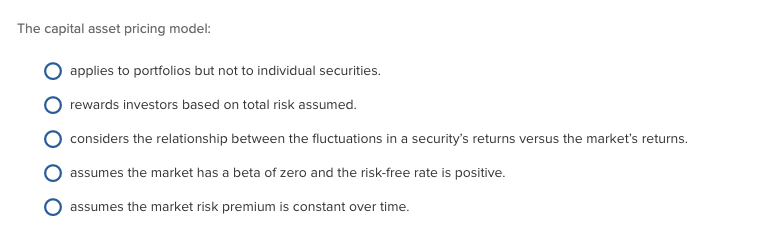The capital asset pricing model: applies to portfolios but not too individual securities. rewards investors based on total risk assumed considers the relationship between the fluctuations in a security's returns versus the market's returns assumes the market has a beta of zero and the risk-free rate is positive. assumes the market risk premium is constant over time.

• ### Explain your answer in depth - show formulas and all relevant stuff The current market price of a security is \$50, the security's expected return is 15%, the riskless rate of interest is 2%, and...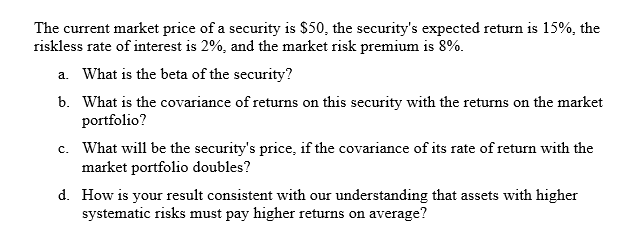Explain your answer in depth - show formulas and all relevant stuff The current market price of a security is \$50, the security's expected return is 15%, the riskless rate of interest is 2%, and the market risk premium is 8% What is the beta of the security? What is the covariance of returns on this portfolio? What will be the security's price, if the covariance of its rate of return with the market portfolio doubles? How is your result...

• ### In the following table, indicate whether each statement refers to the Capital Market Line (CML) or...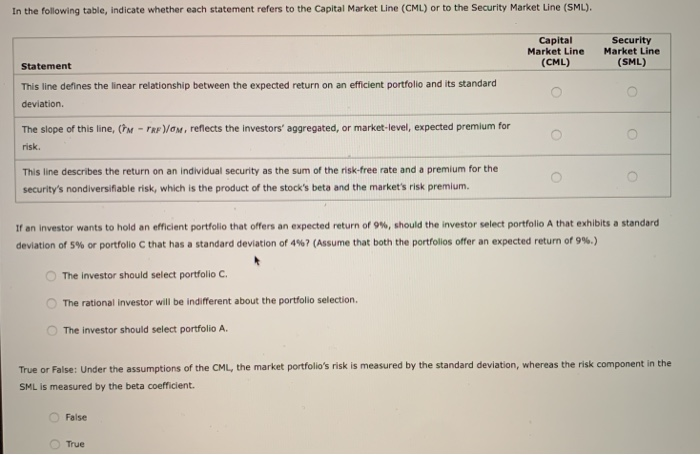In the following table, indicate whether each statement refers to the Capital Market Line (CML) or to the Security Market Line (SML). Capital Market Line (CML) Security Market Line (SML) Statement This line defines the linear relationship between the expected return on an efficient portfolio and its standard deviation The slope of this line, th risk. - TRP/OM, reflects the investors' aggregated, or market-level, expected premium for This line describes the return on an individual security as the sum of...